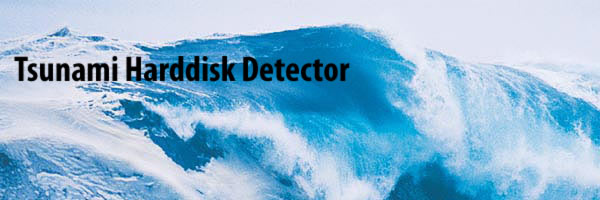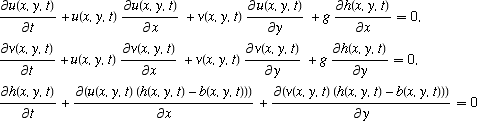Tsunami Harddisk Detector the worlds first freely available P2P warning system[ background ] A tsunami can be generated by any disturbance that rapidly displaces a large mass of water, such as an earthquake, volcanic eruption, landslide or meteorite impact. However, the most common cause is an undersea earthquake. An earthquake which is too small to create a tsunami by itself may trigger an undersea landslide quite capable of generating a tsunami. Waves are formed as the displaced water mass moves under the influence of gravity to regain its equilibrium and radiates across the ocean like ripples on a pond. If the initial event is sensed, the coupled system of partial differential equationscan be utilized to simulate the propagation of tsunami waves and issue a tsunami warning, if necessary. The equations are known as the shallow water wave equations (Pelinovsky et al. 2001, Layton 2002). Here, u and v are the horizontal velocity components of the water surface, x and y are the spatial coordinates of the wave, t is elapsed time, g is the acceleration due to gravity, and h is the height of the wave above the ocean floor topography b. The critical problem is how to sense the initial earthquake. How the Tsunami-Harddisk-Detector copes with these problems is explained here.